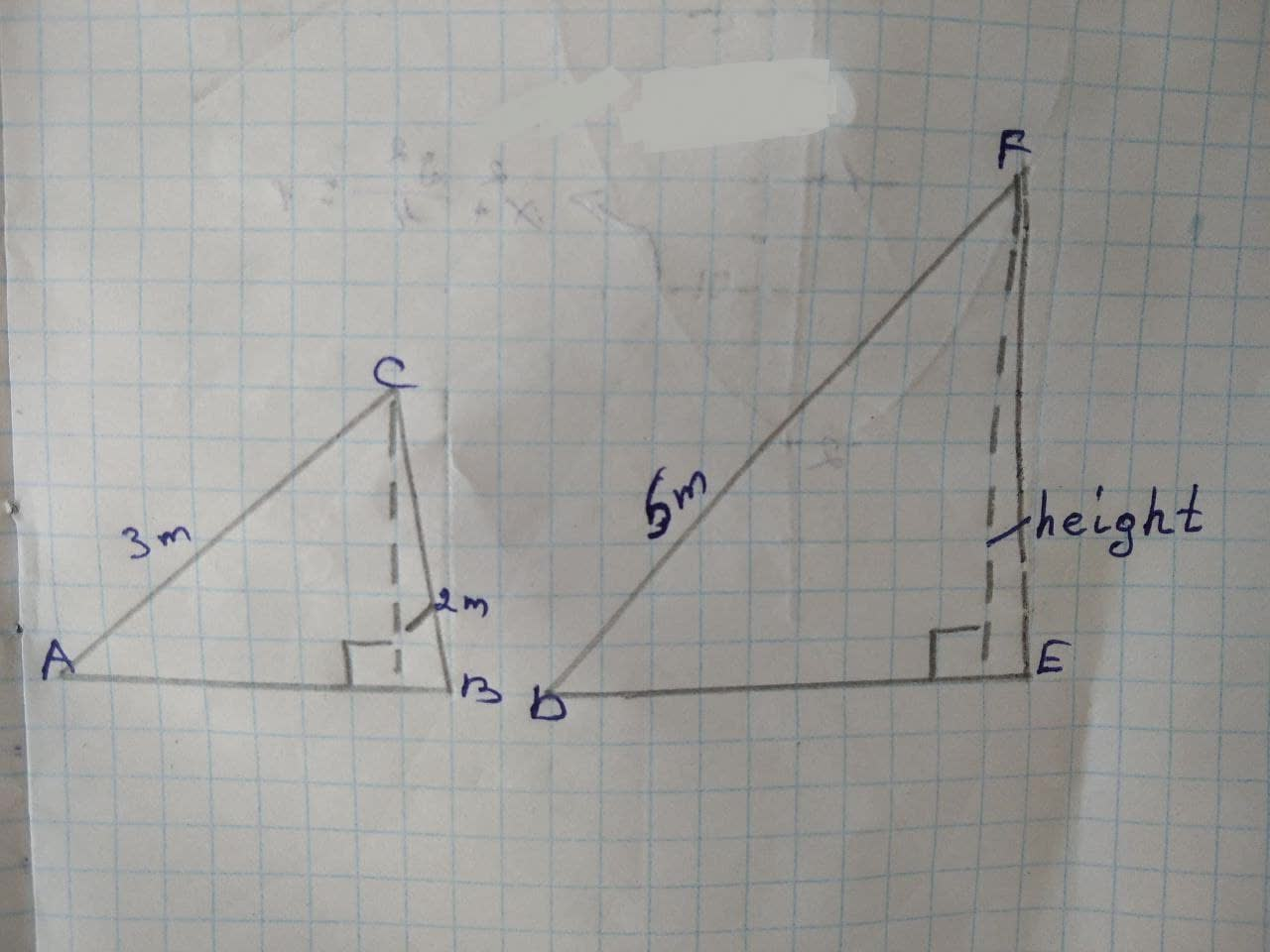## Triangles ABC and DEF are similar triangles.

TrigonometryTriangles ABC and DEF are similar triangles.9 months ago
Triangles ABC and DEF are similar triangles. Solve. Round to the nearest tenth. Find the height of triangle DEF. underline( )m